# Test Metrics in Modelscape

This example shows how to implement various test metrics in MATLAB® using Modelscape™.

For information about test metrics from the model developer's or validator's point of view, see Credit Scorecard Validation Metrics or Fairness Metrics in Modelscape.

### Write Test Metrics

The basic building block of Modelscape metrics framework is the `mrm.data.validation.TestMetric` class. This class defines the following properties:

• `Name`: a human-readable name for the test metric.

• `ShortName`: a concise name for accessing metrics in `MetricsHandler` objects. This name must be a valid MATLAB property name.

• `Value`: the value(s) carried by the metric. The values can be a scalar or a row vector of doubles.

• `Keys`: an n-by-m array of strings that parametrize the values of the metric. m is the length of `Value`. The keys default to an empty string.

• `KeyNames`: a vector of strings of size of the height of `Keys`. It defaults to "Key".

• `Diagnostics`: a free form struct carrying any diagnostics related to the calculation of the metric.

Any subclass of `TestMetric` must implement a constructor and a `compute` method to fill in these values.

For example, the Modelscape statistical parity difference (SPD) metric for bias detection has `Name` "Statistical Parity Difference" and `ShortName` "StatisticalParityDifference". The following table shows how the `Keys` and `KeyNames` are arranged.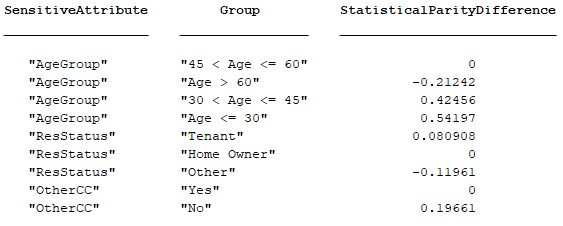Here "SensitiveAttribute" and "Group" are the `KeyNames`, and the two columns with certain attribute-group combinations are the `Keys.` The `ShortName` appears as the third header, and the third column of the table carries the `Value` of the metric.

The base class has the following overridable methods:

• `ComparisonValue``(this)`: use this method to change the value against which thresholds are compared - for example, in statistical hypothesis testing, this should return the p-value associated to the computed statistic.

• `formatResult``(this):` returns by default a table as shown above for the SPD metric.

• `project(this):` returns a restriction of a (non-scalar) metric to a subset of keys. Extend the default implementation in a subclass to cover any diagnostic or auxiliary data carried by the subclass objects.

#### Write Metrics With Visualizations

To write test metrics equipped with visualizations, the metrics should inherit from `mrm.data.validation.TestMetricWithVisualization`. This class adds an additional requirement to the `TestMetric` base class to implement a visualization method with the signature `fig = visualize(this, options).` `options` allows for any name value arguments that may be useful for the given metric. For example, use a particular sensitive attribute with the `StatisticalParityDifference` metric for visualization.

```spdFig = visualize(spdMetric, "SensitiveAttribute","ResStatus"); ```#### Write Metrics Projecting onto Selected Keys

The visualization above shows the SPD metrics for the `ResStatus` attribute only. This plot uses the `project` method of the `TestMetric` class that uses selected keys of a metric. For a metric with N key names, `project` accepts an array of up to N strings as the `Keys` argument. The output restricts the metric to those keys where the first key matches the first element of the array, the second key matches the second element of the array, and so on.

```spdResStatus = project(spdMetric, "Keys", "ResStatus") ```

returns: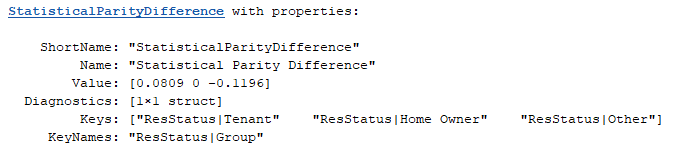On specifying both keys, the results is a scalar metric:

```spdTenant = project(spdMetric, "Keys", ["ResStatus", "Tenant"]) ```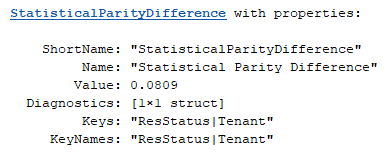The base class implementation of `project` does not handle diagnostics or other auxiliary data carried by the subclass. If necessary, implement this in the subclass using the secondary `keySelection` output in `project`.

#### Write Summarizable Metrics

Summary metrics reveal a different aspect of non-scalar metrics. In the case of the SPD metric, across all the attribute-group pairs, the "summary" SPD value is the value with the largest deviation from the completely non-biased value of zero.

```spdSummary = summary(spdMetric) ```

returns: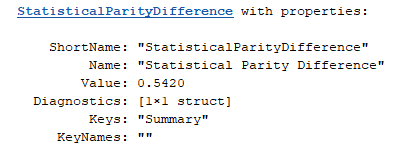Summarize a given TestMetric class by inheriting from `mrm.data.validation.TestMetricWithSummaryValue` class and implementing the abstract `summary` method. This returns a metric of the same type with a singleton `Value`. The meaning of the summary value - if it exists- depends on the metric, so there is no default implementation for this method. However, the protected `summaryCore` method in `TestMetricWithSummaryValue` may be helpful.

### Write Test Thresholds

Test metrics are often compared against thresholds to qualitatively assess of the inputs. For example, a model validator might require that the area under ROC curve should be at least 0.8 for the model to be deemed acceptable, values under 0.7 are red flags, and values between 0.7 and 0.8 require a closer look.

Use Modelscape class `mrm.data.validation.TestThresholds `to implement these thresholds. Encode the thresholds and classifications into a `TestThresholds` object.

```aurocThresholds = mrm.data.validation.TestThresholds([0.7, 0.8], ["Fail", "Undecided", "Pass"]); ```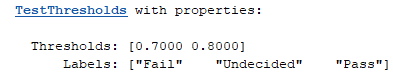These thresholds and labels govern the output of the `status` method of `TestThresholds.` For example, `status(aurocThresholds, 0.72)` returns the following.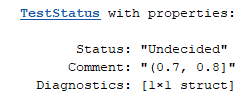`Comment` indicates the interval to which the given input belongs.

#### Customize Thresholds

Implement thresholding regimes, with different narrative strings as Comments, or different diagnostics, as subclasses of `mrm.data.validation.TestThresholdsBase`. Implement the `status` method of the class to populate the `Comment` and `Diagnostics` properties as required.

### Write Statistical Hypothesis Tests

In some cases, notably in statistical hypothesis testing, the relevant quantity to compare against test thresholds is the associated p-value (under some relevant null hypothesis). In these cases, use the test metric class to override the `ComparisonValue` method and return the p-value instead of the `Value` of the metric. For an example, see the Modelscape implementation of the Augmented Dickey-Fuller test.

```edit mrm.data.validation.timeseries.ADFMetric ```

Set the thresholds against which to compare the p-values.

```adfThreshold = mrm.data.validation.PValueThreshold(0.05) ```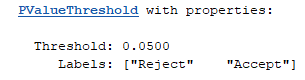This `TestThresholds` object returns `status` as "Reject" for p-values less than 0.05 and "Accept" otherwise.